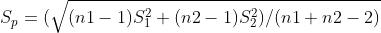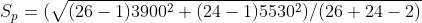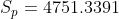##### Question

In: Statistics and Probability

# A researcher claims that the mean of the salaries of elementary school teachers is greater than...

A researcher claims that the mean of the salaries of elementary school teachers is greater than the mean of the salaries of secondary school teachers in a large school district. The mean of the salaries of a random sample of 26 elementary school teachers is $48,250 and the sample standard deviation is$3900. The mean of the salaries of 24 randomly selected secondary school teachers is $45,630 with a sample standard deviation of$5530. At ? = 0.05, can it be concluded that the mean of the salaries of elementary school teachers is greater than the mean of the salaries of secondary school teachers?

## Solutions

##### Expert Solution

To Test :-

H0 :- µ1 = µ2
H1 :- µ1 > µ2

Test Statistic :-
t = (X̅1 - X̅2) / SP √ ( ( 1 / n1) + (1 / n2))t = ( 48250 - 45630) / 4751.3391 √ ( ( 1 / 26) + (1 / 24 ) )
t = 1.948

Test Criteria :-
Reject null hypothesis if t > t(α, n1 + n2 - 2)
Critical value t(α, n1 + n1 - 2) = t( 0.05 , 26 + 24 - 2) = 1.677
t > t(α, n1 + n2 - 2) = 1.948 > 1.677
Result :- Reject Null Hypothesis

Decision based on P value
P - value = P ( t > 1.948 ) = 0.0286
Reject null hypothesis if P value < α = 0.05 level of significance
P - value = 0.0286 < 0.05 ,hence we reject null hypothesis
Conclusion :- Reject null hypothesis

There is sufficient evidence to concluded that the mean of the salaries of elementary school teachers is greater than the mean of the salaries of secondary school teachers.

## Related Solutions

##### A researcher claims that the variation in the salaries of elementary school teachers is greater than...
A researcher claims that the variation in the salaries of elementary school teachers is greater than the variation in the salaries of secondary school teachers. A random sample of the salaries of 30 elementary school teachers has a variance of $8,208, and a random sample of the salaries of 30 secondary school teachers has a variance of$3,817. At α = 0.05, can the researcher conclude that the variation in the elementary school teachers’ salaries is greater than the variation...
##### Salaries for teachers in a particular elementary school district are normally distributed with a mean of...
Salaries for teachers in a particular elementary school district are normally distributed with a mean of $44,000 and a standard deviation of$6,500. We randomly survey ten teachers from that district. 1.Find the probability that the teachers earn a total of over $400,000 2.If we surveyed 70 teachers instead of ten, graphically, how would that change the distribution in part d? 3.If each of the 70 teachers received a$3,000 raise, graphically, how would that change the distribution in part...
##### Salaries for teachers in a particular elementary school district are normally distributed with a mean of...
Salaries for teachers in a particular elementary school district are normally distributed with a mean of $44,000 and a standard deviation of$6,500. We randomly survey ten teachers from that district. Find the 85th percentile for the sum of the sampled teacher's salaries to 2 decimal places.
##### : A researcher is interested in whether salaries for middle school teachers were less than salaries...
: A researcher is interested in whether salaries for middle school teachers were less than salaries for nurses in Arkansas. A statewide salary survey is conducted using random sampling. The Data Analysis output for the various tests used when comparing two group means are shown below. The significance level was .05. F-Test Two-Sample for Variances Teachers Nurses Mean 45946.07 53365.13 Variance 68256753 86820446 Observations 300 300 df 299 299 F 0.7862 P(F<=f) one-tail 0.0190 F Critical one-tail 0.8265 t-Test: Paired...